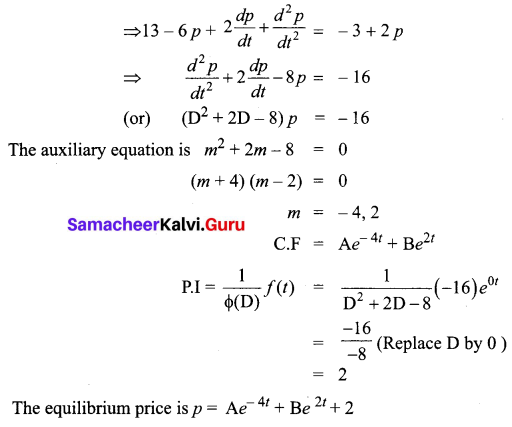# Samacheer Kalvi 12th Business Maths Solutions Chapter 4 Differential Equations Ex 4.5

Students can download 12th Business Maths Chapter 4 Differential Equations Ex 4.5 Questions and Answers, Samacheer Kalvi 12th Business Maths Book Solutions Guide Pdf helps you to revise the complete Tamilnadu State Board New Syllabus and score more marks in your examinations.

## Tamilnadu Samacheer Kalvi 12th Business Maths Solutions Chapter 4 Differential Equations Ex 4.5

Solve the following differential equations:

Question 1.
$$\frac{d^{2} y}{d x^{2}}-6 \frac{d y}{d x}+8 y=0$$
Solution:
Given (D2 – 6D + 8) y = 0, D = $$\frac{d}{d x}$$
The auxiliary equations is
m2 – 6m + 8 = 0
(m – 4)(m – 2) = 0
m = 4, 2
Roots are real and different
The complementary function (C.F) is (Ae4x + Be2x)
The general solution is y = Ae4x + Be2x

Question 2.
$$\frac{d^{2} y}{d x^{2}}-4 \frac{d y}{d x}+4 y=0$$
Solution:
The auxiliary equations A.E is m2 – 4m + 4 = 0
(m – 2)2 = 0
m = 2, 2
Roots are real and equal
The complementary function (C.F) is (Ax + B) e2x
The general solution is y = (Ax + B) e2x

Question 3.
(D2 + 2D + 3) y = 0
Solution:
The auxiliary equations A.E is m2 + 2m + 3 = 0
⇒ m2 + 2m + 1 + 2 = 0
⇒ (m + 1)2 = -2
⇒ m + 1 = ± √2i
⇒ m = – 1 ± √2i
It is of the form α ± iβ
The complementary function (C.F) = e-x [A cos √2 x + B sin √2 x]
The general solution is y = e-x [A cos √2 x + B sin √2 x]

Question 4.
$$\frac{d^{2} y}{d x^{2}}-2 k \frac{d y}{d x}+k^{2} y=0$$
Solution:
Given (D2 – 2kD + k2)y = 0, D = $$\frac{d}{d x}$$
The auxiliary equations is m2 – 2km + k = 0
⇒ (m – k)2 = 0
⇒ m = k, k
Roots are real and equal
The complementary function (C.F) is (Ax + B) ekx
The general solution is y = (Ax + B) ekx

Question 5.
(D2 – 2D – 15) y = 0 given that $$\frac{d y}{d x}$$ = 0 and $$\frac{d^{2} y}{d x^{2}}$$ = 2 when x = 0
Solution:
A.E is m2 – 2m – 15 = 0
(m – 5)(m + 3) = 0
m = 5, -3
C.F = Ae5x + Be-3x
The general solution is y = Ae5x + Be-3x …….. (1)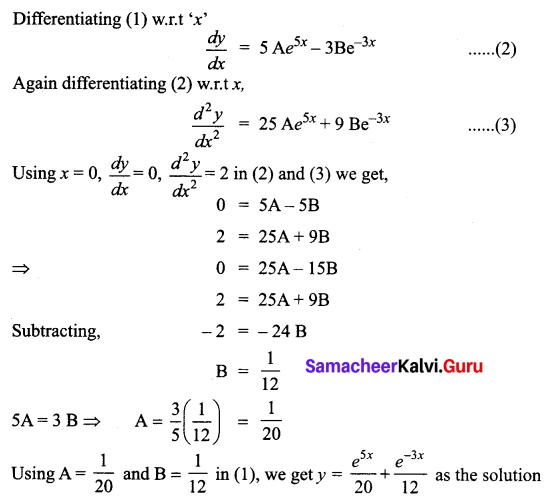Question 6.
(4D2 + 4D – 3) y = e2x
Solution:
The auxiliary equations is 4m2 + 4m – 3 = 0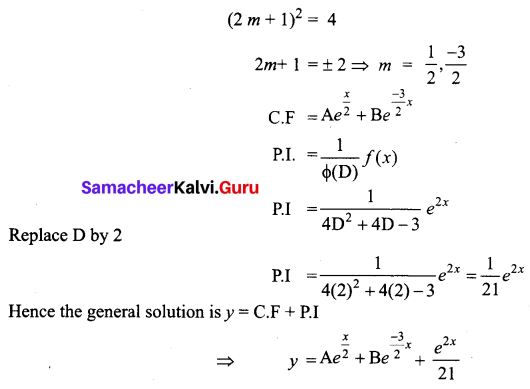Question 7.
$$\frac{d^{2} y}{d x^{2}}$$ + 16y = 0
Solution:
Given (D2 + 16) y =0
The auxiliary equation is m2 + 16 = 0
⇒ m2 = -16
⇒ m = ± 4i
It is of the form α ± iβ, α = 0, β = 4
The complementary function (C.F) is e0x [A cos 4x + B sin 4x]
The general solution is y = [A cos 4x + B sin 4x]

Question 8.
(D2 – 3D + 2)y = e3x which shall vanish for x = 0 and for x = log 2
Solution:
A.E is m2 – 3m + 2 = 0
⇒ (m – 2) (m – 1) = 0
⇒ m = 2, 1
CF = Ae2x + Bex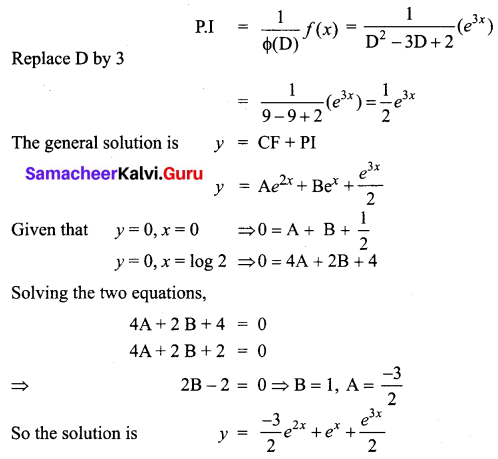Question 9.
(D2 + D – 6)y = e3x + e-3x
Solution:
A.E is m2 + m – 6 = 0
(m + 3) (m – 2) = 0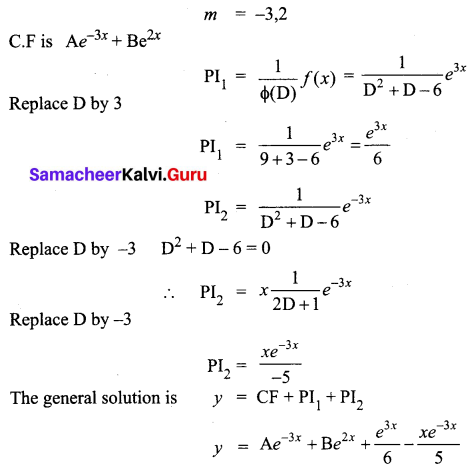Question 10.
(D2 – 10D + 25)y = 4e5x + 5
Solution:
A.E is m2 – 10m + 25 = 0
⇒ (m – 5)2 = 0
⇒ m = 5, 5
C.F = (Ax + B) e5x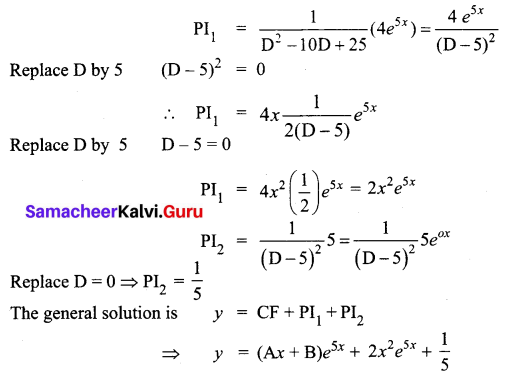Question 11.
(4D2 + 16D + 15) y = 4$$e^{\frac{-3}{2} x}$$
Solution:
A.E is 4m2 + 16m + 15 = 0
(2m + 3) (2m + 5) = 0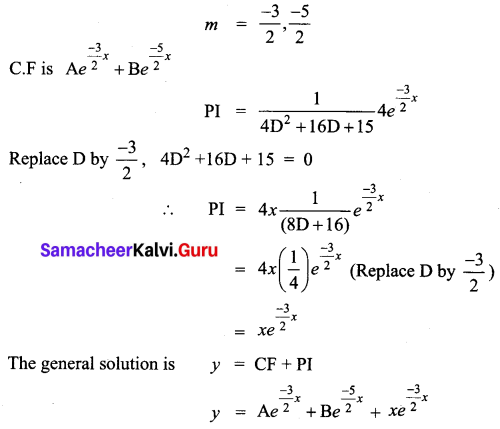Question 12.
(3D2 + D – 14)y = 13e2x
Solution: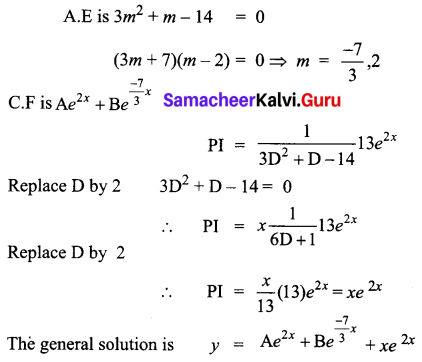Question 13.
Suppose that the quantity demanded Qd = 13 – 6p + 2$$\frac{d p}{d t}+\frac{d_{2} p}{d t^{2}}$$ and quantity supplied Qs = -3 + 2p where p is the price. Find the equilibrium price for market clearance.
Solution:
For market clearance, the required condition is Qd = Qs